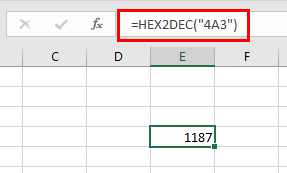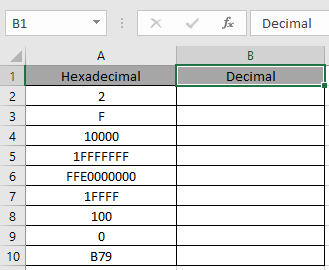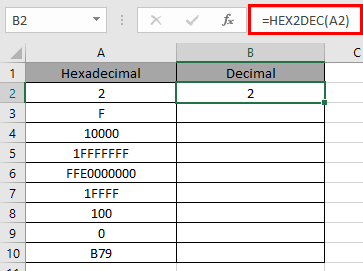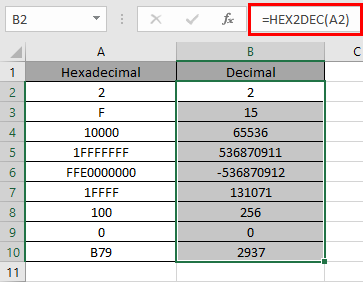# How to use the HEX2DEC Function in Excel

In this article, we will learn about how to use the HEX2DEC function in Excel.

HEX2DEC function in excel is used to convert Hexadecimal representation of numbers of radix 16 to Decimal numbers (radix = 10).

The table shown below shows you some of the most used base & their radix of Alpha - numeric characters

 Base radix Alpha-Numeric Characters Binary 2 0 - 1 Octal 8 0 - 7 Decimal 10 0 - 9 hexadecimal 16 0 - 9 & A - F hexatridecimal 36 0 - 9 & A - Z

Hexadecimal number is representation of a number of radix 16. 10 digits starting from 0, 1, 2, 3, 4, 5, 6, 7, 8 ,9 along with 6 alphabets starting from A, B, C, D, E & F where Decimal number representation have 8 digits from 0, 1, 2, 3, 4, 5, 6, 7, 8, 9. The below table will help you understand better.

 Decimal hexadecimal 0 0 1 1 2 2 3 3 4 4 5 5 6 6 7 7 8 8 9 9 10 A 11 B 12 C 13 D 14 E 15 F

Conversion formulation is shown below:

4A3

1. Separate the digits
2. Multiply the separated digit with 160, 161,162, 16and so on starting from the rightmost digit correspondingly.
3. Get the sum of all the multiplied numbers. There it is our decimal number
 4 A ( = 10 decimal) 3 4 x 162 10 x 161 3 x 160 1024 160 3 4A3 ( octal ) = 1187( decimal )

The HEX2DEC function converts the Hexadecimal number of radix 16 to the Decimal number of radix 10.
Syntax:

=HEX2DEC ( number, [places] )

Number : Hexadecimal number which returns the 10 character Decimal number.
[Places] : [optional] number where result expressed upto the number.
The input value to the function cannot contain more than 10 characters ( 10 bits ). Where first number returns the sign of the number (positive or negative) & Other 9 returns the value after the sign.To get a negative number, input number must have 10 characters starting with first bit as it represents the negative sign. The negative number is calculated using two's complement notation. Any less than 10 characters, the function considers default 0s in front.

Now let’s get more understanding of the function via using them in some examples.Here we have some Hexadecimal values in Hexadecimal Column. We need to convert these Hexadecimal numbers to Decimal value.

Use the formula in Hexadecimal column:

=HEX2DEC (A2)

A2 : number provided to the function as cell reference or text in quotes

Values to the HEX2DEC function is provided as cell reference.The HEX2DEC representation of 2 of base 16 (hexadecimal) is 2 of base 10 (decimal).

216= 210

Now copy the formula to other cells using the Ctrl + D shortcut key.As you can see here the HEX2DEC function returns the result of the input values.

Notes:

1. Numbers can be given as argument to the function directly with quotes or cell reference in excel.
2. The function doesn’t consider the [places] number in case of a negative decimal number.
3. If the input Hexadecimal number is negative it cannot be less than 1FFFFFFF and if the number is positive it cannot be greater than FFE0000000 .
4. If the input [places] number is not an integer, it is truncated by the function.
5. The function returns the Decimal value for the max value of + 536870911 & minimum value upto - 536870912 (only integers).
6. The function returns the #NUM! Error
1. If the input Hexadecimal number is less than 1FFFFFFF or greater than FFE0000000 .
2. If the input number [places] is zero or negative.
3. If the input number [places] is not sufficient for the resulting positive Decimal number.
7. The function returns the #VALUE! Error
1. If the input [places] number is text or non-numeric.

Hope you understood how to use HEX2DEC function and referring cell in Excel. Explore more articles on Excel mathematical conversion functions here. Please feel free to state your query or feedback for the above article.

Related Articles:

How to use the DEC2BIN Function in Excel

How to use the BIN2OCT Function in Excel

How to use the BIN2HEX Function in Excel

How to use the DEC2OCT Function in Excel

How to use the DEC2HEX Function in Excel

How to use the OCT2BIN Function in Excel

How to use the OCT2DEC Function in Excel

How to use the OCT2HEX Function in Excel

How to use the HEX2BIN Function in Excel

How to use the HEX2OCT Function in Excel

How to use the HEX2DEC Function in Excel

Popular Articles:

If with wildcards

Vlookup by date

Join first and last name in excel

Count cells which match either A or B

Terms and Conditions of use

The applications/code on this site are distributed as is and without warranties or liability. In no event shall the owner of the copyrights, or the authors of the applications/code be liable for any loss of profit, any problems or any damage resulting from the use or evaluation of the applications/code.